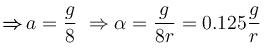Courses

# Rigid Body Dynamics NAT Level - 2

## 10 Questions MCQ Test Topic wise Tests for IIT JAM Physics | Rigid Body Dynamics NAT Level - 2

Description
This mock test of Rigid Body Dynamics NAT Level - 2 for Physics helps you for every Physics entrance exam. This contains 10 Multiple Choice Questions for Physics Rigid Body Dynamics NAT Level - 2 (mcq) to study with solutions a complete question bank. The solved questions answers in this Rigid Body Dynamics NAT Level - 2 quiz give you a good mix of easy questions and tough questions. Physics students definitely take this Rigid Body Dynamics NAT Level - 2 exercise for a better result in the exam. You can find other Rigid Body Dynamics NAT Level - 2 extra questions, long questions & short questions for Physics on EduRev as well by searching above.
*Answer can only contain numeric values
QUESTION: 1

### A carpet of mass M and of inextensible material is rolled along its length in the form of a cylinder of radius R and is kept on a rough floor. The carpet starts unrolling without sliding on the floor where a negligibly small push is given to it. The horizontal velocity of the axis of the cylindrical part of the carpet when its radius reduces to R/2. is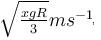then  x  is :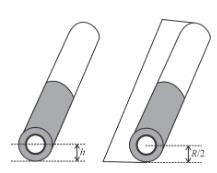Solution: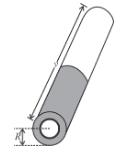ρ = density of carpet material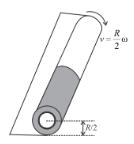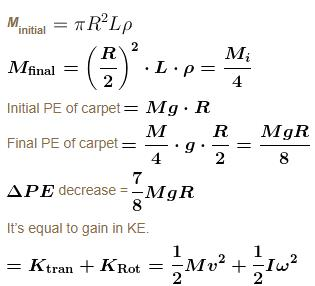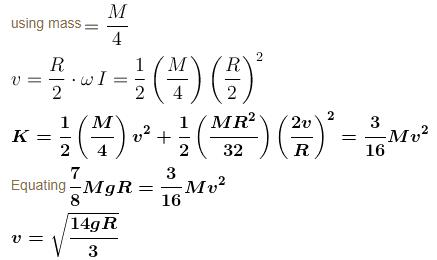*Answer can only contain numeric values
QUESTION: 2

### A thin uniform square plate ABCD of side a and mass m is suspended in vertical plane as shown in the figure. AE and BF  are two massless inextensible strings.  The line AB is horizontal. Find the tension in the string AE just after BF is cut is 2mg/x , then is :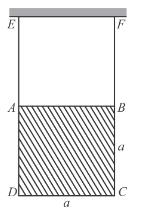Solution:

Let b and α

are linear according to centre of mass and angular according of the plane, just after BF is cut

mg – T = mb         ...(1)

Taking torque about centre of mass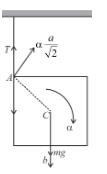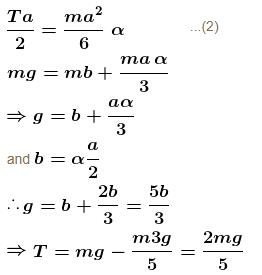*Answer can only contain numeric values
QUESTION: 3

### A uniform rod of length R and mass M is freely to rotate about a horizontal axis passing through hinge P as is figure. First it is taken aside such that it becomes horizontal and then released. At the lowest point the rod hits the block B of mass m and stops. If ratio of mass of rod M to the mass of block m such that the block B completes the circle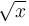then x is. Neglect any friction.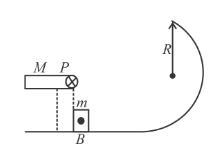Solution:

Minimum velocity required by block m to complete the motion in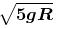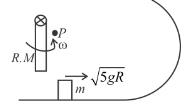conserving mechanical energy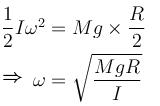conserving angular momentum w.r.t. P before and after collision.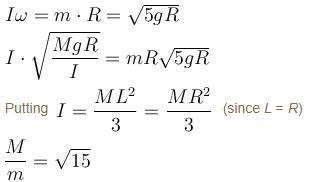*Answer can only contain numeric values
QUESTION: 4

A square plate of edge a/2 is cut out from a uniform square plate of edge a’ as shown in figure. The mass of the remaining portion is M. The moment of inertia of the shaded portion about an axis passing through O (centre of the square of side a and perpendicular to plane of the plate is given by nma2. Find the value of n?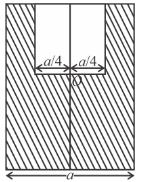Solution:

Let m1 = mass of the square plate of slide a
and m2 = mass of the square of side ‘a/2’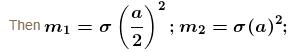(σ being the areal density)
and m2 – m1 = M.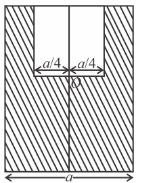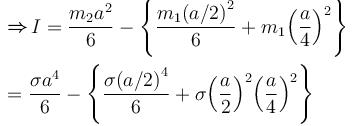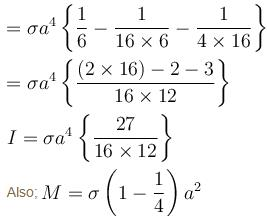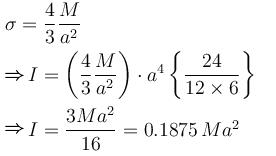*Answer can only contain numeric values
QUESTION: 5

In the figure shown a block B of mass m can slide on a fixed horizontal smooth plane. A uniform solid sphere A of radius r of the same mass rolls without sliding on the block B. Find the angular acceleration of the sphere xg/r then x is :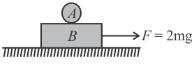Solution: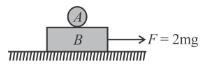The freely body diagrams of sphere A and Block B are as shown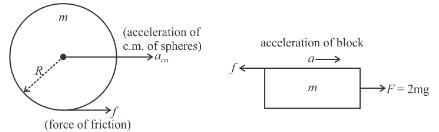Applying Newton’s law to block and sphere

F – f = ma       ...(1)
f = macm     ...(2)
Since the sphere does not slip cover the block, therefore from constraint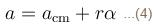solving equation (1), (2), (3) and (4)
we get the angular acceleration of sphere

*Answer can only contain numeric values
QUESTION: 6

A billiard ball at rest is struck horizontally one tenth of the diameter below the top. If P be the linear impulse of the blow find the initial kinetic energy of the ball is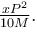Then x is given by the mass of the ball is being M.

Solution: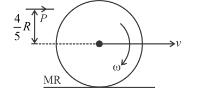Using momentum equation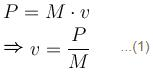using angular impulse-momentum equation. w.r.t. centre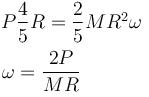Total K.E. = Translational K.E. + Rotational K.E.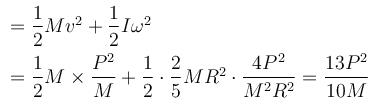*Answer can only contain numeric values
QUESTION: 7

A uniform rod of length 75 cm hinged at one ends and is free to rotate in vertical plane. It is released from rest when rods is horizontal. When the rod becomes vertical, it is broken at mid-point and lower part now moves freely. The distance of centre of lower part from it again becomes vertical for the first time is  r. Find the approximate value of  2r.

Solution: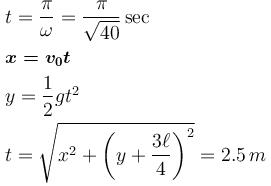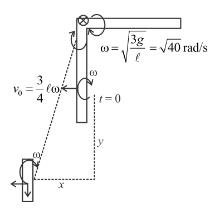*Answer can only contain numeric values
QUESTION: 8

Figure shows an arrangement of masses hanging from a ceiling. In equilibrium, each rod is horizontal, has negligible mass and extends three times as far to the right of the wire supporting it as to the left. If mass m4, is 48 kg then mass m1 (in kg) is equal to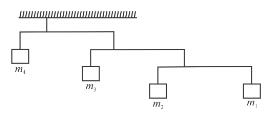Solution: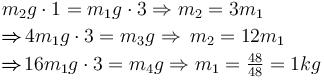*Answer can only contain numeric values
QUESTION: 9

A solid homogeneous cylinder of height h = 1m and base r = 1m  is kept vertically on a conveyor belt  moving horizontally with an increasing velocity v = 1 + t2. If the cylinder is not allowed to slip find the time when the cylinder is about to topple.

Solution:

WRT of belt, pseudo force ma act on cylinder at centre of mass as shown about to cylinder will be just about to topple when torque to weight w.r.t. P.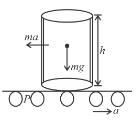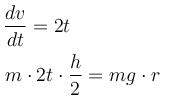*Answer can only contain numeric values
QUESTION: 10

A small block of mass m is rigid attached at P to a ring of mass 3m and radius r. The system is released from rest at θ = 90° and rolls without sliding. The angular acceleration of hoop just after released is given by ng/r.  Find the value of n.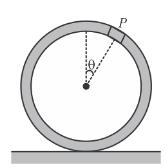Solution:

f = 4a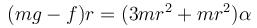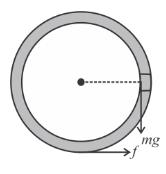mg – f = 4ma
from (1) and (2
⇒ 8ma = mg